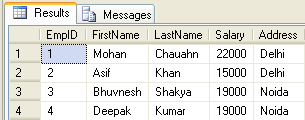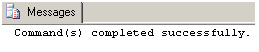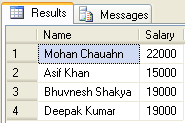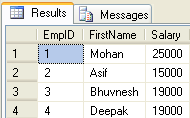# Different Types of SQL Server Functions

Function is a database object in SQL Server. Basically, it is a set of SQL statements that accept only input parameters, perform actions and return the result. Function can return an only single value or a table. We can’t use a function to Insert, Update, Delete records in the database table(s). For more about stored procedure and function refer to the article Difference between Stored Procedure and Function

1. ## System Defined Function

These functions are defined by SQL Server for a different purpose. We have two types of system defined function in SQL Server

1. ## Scalar Function

Scalar functions operate on a single value and return a single value. Below is the list of some useful SQL Server Scalar functions.

System Scalar Function
Scalar Function
Description
abs(-10.67)
This returns an absolute number of the given number means 10.67.
rand(10)
This will generate a random number of 10 characters.
round(17.56719,3)
This will round off the given number to 3 places of decimal means 17.567
upper('dotnet')
This will returns the upper case of given string means 'DOTNET'
lower('DOTNET')
This will returns the lower case of given string means 'dotnet'
ltrim(' dotnet')
This will remove the spaces from the left-hand side of 'dotnet' string.
convert(int, 15.56)
This will convert the given float value to integer means 15.

2. ## Aggregate Function

Aggregate functions operate on a collection of values and return a single value. Below is the list of some useful SQL Server Aggregate functions.

System Aggregate Function
Aggregate Function
Description
max()
This returns maximum value from a collection of values.
min()
This returns the minimum value from a collection of values.
avg()
This returns an average of all values in a collection.
count()
This returns no of counts from a collection of values.

2. ## User Defined Function

These functions are created by the user in the system database or in a user-defined database. We three types of user-defined functions.

1. ## Scalar Function

The user-defined scalar function also returns a single value as a result of actions performed by the function. We return any datatype value from a function.

``` --Create a table
CREATE TABLE Employee
(
EmpID int PRIMARY KEY,
FirstName varchar(50) NULL,
LastName varchar(50) NULL,
Salary int NULL,
)
--Insert Data
--See created table
Select * from Employee `````` --Create function to get emp full name
Create function fnGetEmpFullName
(
@FirstName varchar(50),
@LastName varchar(50)
)
returns varchar(101)
As
Begin return (Select @FirstName + ' '+ @LastName);
end `````` --Calling the above created function
Select dbo.fnGetEmpFullName(FirstName,LastName) as Name, Salary from Employee ```2. ## Inline Table-Valued Function

The user-defined inline table-valued function returns a table variable as a result of actions performed by the function. The value of the table variable should be derived from a single SELECT statement.

``` --Create function to get employees
Create function fnGetEmployee()
returns Table
As
return (Select * from Employee) `````` --Now call the above created function
Select * from fnGetEmployee() ```3. ## Multi-Statement Table-Valued Function

A user-defined multi-statement table-valued function returns a table variable as a result of actions performed by the function. In this, a table variable must be explicitly declared and defined whose value can be derived from multiple SQL statements.

``` --Create function for EmpID,FirstName and Salary of Employee
Create function fnGetMulEmployee()
returns @Emp Table
(
EmpID int,
FirstName varchar(50),
Salary int
)
As
begin
Insert into @Emp Select e.EmpID,e.FirstName,e.Salary from Employee e;
--Now update salary of first employee
update @Emp set Salary=25000 where EmpID=1;
--It will update only in @Emp table not in Original Employee table
return
end `````` --Now call the above created function
Select * from fnGetMulEmployee() `````` --Now see the original table. This is not affected by above function update command
Select * from Employee ```#### Note

1. Unlike Stored Procedure, Function returns an only single value.

2. Unlike Stored Procedure, Function accepts only input parameters.

3. Unlike Stored Procedure, Function is not used to Insert, Update, Delete data in a database table(s).

4. Like Stored Procedure, Function can be nested up to 32 levels.

5. User Defined Function can have upto 1023 input parameters while a Stored Procedure can have upto 2100 input parameters.

6. User Defined Function can't return XML Data Type.

7. User Defined Function doesn't support Exception handling.

8. User Defined Function can call only Extended Stored Procedure.

9. User Defined Function doesn't support set options like set ROWCOUNT etc.

##### Summary